# Problem 54735. Solve an ODE: concentrations predicted by the cells-in-series model

One approach for predicting mixing and transport of contaminants in a river is the cells-in-series model. The model divides a river into several well-mixed cells of volume V. Then if the discharge (i.e., the volume of water flowing past a cross section per unit time) is Q and the first-order decay coefficient (dimensions of time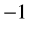) is k, the concentration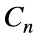in the nth cell is given byWrite a function to compute the maximum concentration in the nth cell and the time it occurs assuming that the concentration in the first cell (i.e.,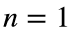) is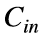at time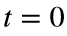and no contaminant enters the first cell from upstream.

### Solution Stats

100.0% Correct | 0.0% Incorrect
Last Solution submitted on Jun 24, 2022

### Community Treasure Hunt

Find the treasures in MATLAB Central and discover how the community can help you!

Start Hunting!